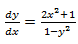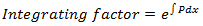Goseeko blog# What are linear differential equations?

## Overview

An equation consisting of a differential coefficient is called a differential equation.

LDE has many applications in engineering problems.

For example-is the differential equation.

A differential equation of the form

is known as a linear differential equation or simply LDE.

P and Q, are functions of x or constants.

For example-

The solution of LDE is-

Note

1.2.     If the RHS of LDE is zero for all x then it is said to be homogeneous, otherwise non-homogeneous.

## Solving a linear differential equation

1. First change the given equation to the standard form of LDE, which is

2. Find the integrating factor

3. Then the solution of LDE is-

## Solved examples

Example: Solve

Solution-

First we will convert the given equation in standard LDE form-

Where Q = sin x and P = 2/x

Now we will find the integrating factor-

Then the solution is-

Integrating by parts-

Example: Solve-

Solution-

The given equation is already in the form of standard LDE.

Now we will find the IF-

So that the solution is-